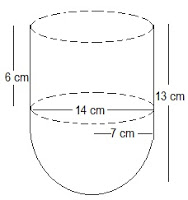Newbie

# A vessel is in the form of a hollow hemisphere mounted by a hollow cylinder. The diameter of the hemisphere is 14 cm and the total height of the vessel is 13 cm. Find the inner surface area of the vessel..Q.2

• 0

In chapter surface areas and volumes of ncert class 10 its very hard to solve the formulas .What is the formula of this question exercise 13.1 question no.2, give me the simplest method of this question its very tricky question, please help me to solve this question in a easiest and best way.A vessel is in the form of a hollow hemisphere mounted by a hollow cylinder. The diameter of the hemisphere is 14 cm and the total height of the vessel is 13 cm. Find the inner surface area of the vessel..

Share

1. The diagram is as follows:Now, the given parameters are:

The diameter of the hemisphere = D = 14 cm

The radius of the hemisphere = r = 7 cm

Also, the height of the cylinder = h = (13-7) = 6 cm

And, the radius of the hollow hemisphere = 7 cm

Now, the inner surface area of the vessel = CSA of the cylindrical part + CSA of hemispherical part

(2πrh+2πr2) cm2 = 2πr(h+r) cm2

2×(22/7)×7(6+7) cm2 = 572 cm2

• 0